# GE Pro v0.7a

1. Introduction
2. User Interface overview
3. Tools

## Introduction

GE Pro is win32 program designed for fast preview and preprocessing of spectral data.
Multiple ASCII data files can be loaded and processed. The structure of these files should be one of the following:
1. single Y column (in this case number of row will be used as X)
2. one X and Y column
3. one X and multiple Y column
files may content comment strings starting with ".

Example of ASCII file :
"any comment here
1.5 0
2.5 1
3.5 4
4.5 8

the interface of the program is optimized for maximum performance, for example you need only few seconds to remove constant shelf form 50 spectra. You have to press A (opens Open window), select files, press Add and Ok then press Ctrl-A and C (subtracts constant shelf from all files), press S to save all files.

## User Interface overview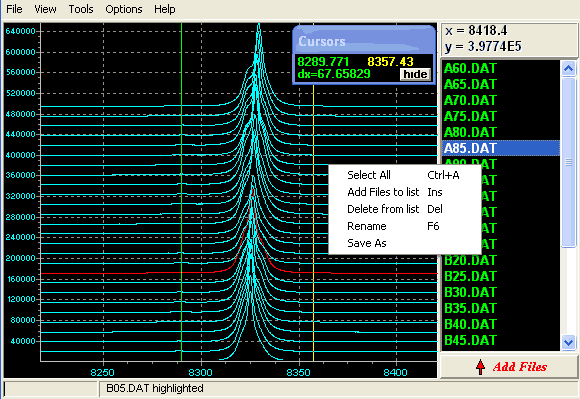The workspace of the program consists of two major regions: graphs of data and list of loaded files (to the right). Each graph corresponds to certain file in the list and highlighted when file is selected. You can mark multiple files in the list holding CTRL or SHIFT to process them simultaneously (you can press Ctrl-A to mark all files). You can change name of file by pressing F6 (this won't result in changed name of original file, this name will be used when saving processed files)

You can easily pan by holding right mouse button and dragging field of view. To zoom into some region select it holding left mouse button and moving it from up-left to down-right. To zoom out press R or press left mouse button and move it up-left. You can also use + and - to zoom in and out and PgUp or PgDown to increase and decrease vertical scale.

There are two cursors(yellow and green)in the graph window (initially parked to to the left). Positions of each cursor and distance between them shown in cursor window. To move yellow cursor use keyboard arrows, to move green one use SHIFT+ARROWS, holding CTRL accelerates cursor movements. To zoom into between cursors press W.

It's possible to customize color scheme using Options=>Colors

. You can add files to the list by pressing A or choosing File=>Add Files in the program's menu. You will get into Open window: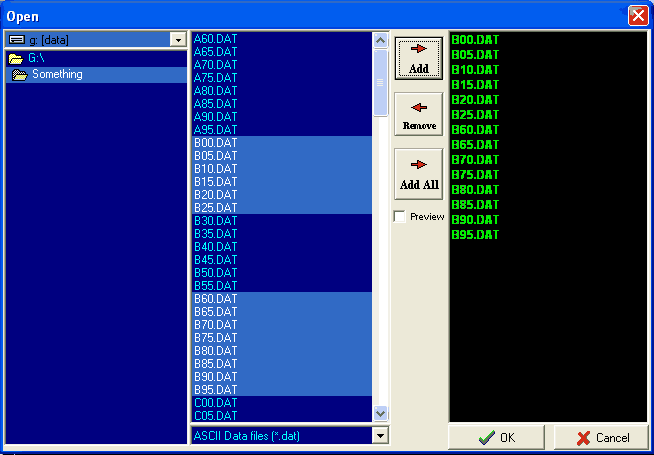here you can browse and select files you want to process. Press Add-> and OK to load them. In this window you also can preview ASCII files by checking preview checkbox.

## Tools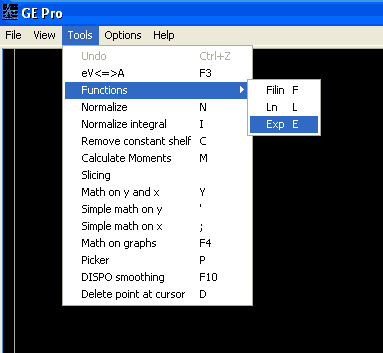Most of the tools will process all marked files.

### eV<=>A

converts electron-volts to angstroms and vice versa.(1eV=8.065544/A)

### Functions

the functions submenu includes three functions :
1) Filin(y) (Filin(y)=1 if y<1 and Filin(y)=y if y>1 )
2) ln(y)
3) exp(y)
you can do the same using Math on y and x tool.

### Normalize

normalizes all selected graphs(makes all graphs equal in height).

### Normalize integral

normalizes integrals of all selected graphs. Integrals are calculated in the field of view.

### Remove constant shelf

this tool finds minimum y value and subtracts it from all y values.

### Calculate moments

calculates Integral(M0), Center of mass(M1), Dispersion and Asymmetry coefficient using formulae: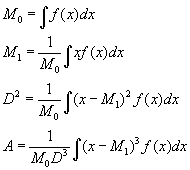All integrals are calculated in the field of view. When processing multiple files programm extracts numbers from filenames and and uses it as X value when saving derived moments(everything is shown in a table). This feature is useful when filename includes a parameter (temperature,angle, etc.)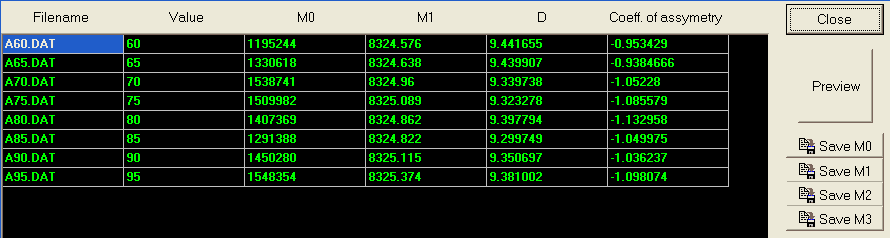### Slicing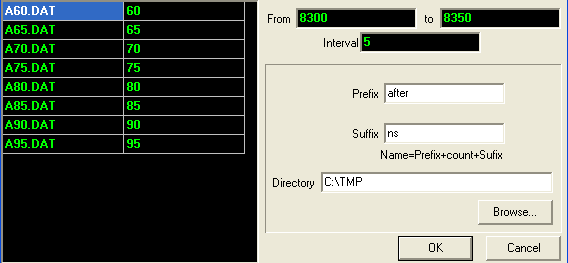This tool 'slices' all marked files. It calculates integrals in intervals from 1st value to 2nd value end forms files that consist of X values extracted from filenames and integrals as Y values. Resulting filenames include value of start of each interval.

### Math on y and x

Here you can enter any equation. Following functions accepted:
^ - Power only works with positive values
exp,ln
cos,sin,tan,ctg,asin,acos
abs,fil(=filin)
Examples of equations:
ln(x)
exp(-((x-50)/200))
12398/x

### Simple math on y

Using this tool you can multiply graph Y values by 1st entered value and add 2nd entered. You can add (or multiply by) 3d value multiplied by Number of graph. (Useful if you want insert gaps between graphs)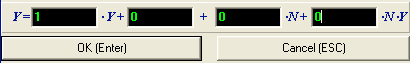### Simple math on x

The same as above but for X values.

### Math on graphs

Here you can add(subtract,divide,multiply by)one graph to all marked graphs. Useful for subtracting background and normalization.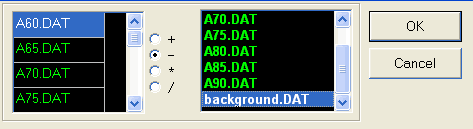### Picker

click mouse over desired point in the graph window to add it to the list.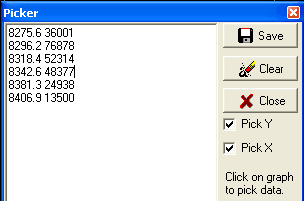### DISPO smoothing

smoothes graphs using 5 points DISPO smoothing.

### Delete point at cursor

deletes point in the selected graph at the yellow cursor.